Blog

# Lab Notes: How We Made Joins 23 Thousand Times Faster, Part One

Even though we target CrateDB at machine data use-cases, where denormalized schemas are common, joining tables can be a powerful tool for sophisticated analytics.

CrateDB has supported joins for a while. We use the nested loop algorithm, and in some instances, a modified version of this that optimizes for distributed query execution.

The nested loop algorithm is relatively simple to implement and was easily adjusted to execute cross joins, left outer joins, right outer joins, and full outer joins. This does however come at a cost.

The nested loop algorithm has a quadratic time complexity. Specifically, O(M * N), where M and N are the number of rows of the two tables joined, which is in turn bounded by O(N2) (N > M). If the joined tables have a high row cardinality, the number of operations required to perform the join increase quadratically, and so does execution time.

Since many CrateDB users want to run joins on large tables for their analytics, we decided to introduce a new join algorithm that would have better join performance than the nested loop algorithm. This work is now complete, and in this post, I will show you how we approached the problem.

Please note: join algorithms and their performance characteristics are common knowledge for RDBMS developers. This miniseries is written for people who want a behind the scenes look as we implement these algorithms and adapt them for CrateDB and our distributed SQL query execution engine.

## Equi-Joins

An equi-join is an inner join where the join condition has a single equality operator (`=`) or multiple equality operators chained together with `AND` operators.

For instance, here's an inner join with a single equality operator:

``````SELECT *
FROM t1
JOIN t2
ON t1.a = t2.b
``````

And here's one with multiple:

``````SELECT *
FROM t1
JOIN t2
ON t1.a = t2.b
AND t1.x = t2.y
AND [...]
``````

In general, equi-joins tend to be the most common type of join. So for this work, we chose to focus specifically on equi-join performance.

## Sorted Merge vs. Hash Join

Two main algorithms can improve on the performance of the nested loop algorithm:

Both of these have pros and cons. So before we continued, we wanted to measure their performance under different workloads and see which one would better fit for typical CrateDB use-cases.

### Hash Joins

The hash join algorithm has two phases:

• Build phase
• Probe phase

During the build phase, a hash map is created. Rows from the left table are inserted into this hash map using a hash that is computed from the value of the join condition.

Consider this query:

``````SELECT *
FROM t1
JOIN t2
ON t1.a = t2.b
``````

In this example, the hash map is populated with rows from `t1` using a hash that is computed from the value of the `a` column.

After the hash map is built, the probe phase starts.

During the probe phase, the hash value corresponding to the join condition is calculated for every row of the inner (right) table. This value is then looked up in the hash map, and for every corresponding row that is found, the matching outer and inner rows are combined and emitted as a single result row.

Here's a visual overview: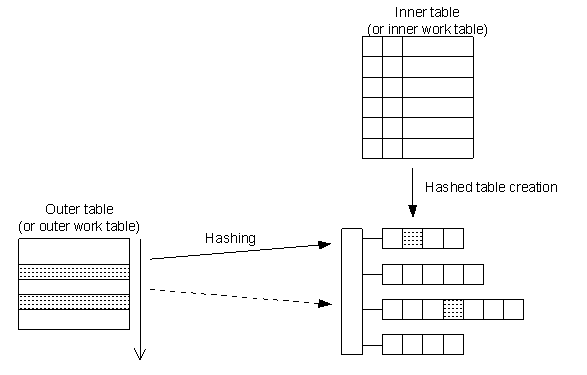So, in summary, the pros:

• Relatively easy implementation (compared to sorted-merge)
• A linear time complexity of O(M + N), which is better than both the nested loop join (quadratic) and sorted merge join (quasilinear) algorithms

And the cons:

• One of the two tables must fit in memory
• Only equivalence operators (`=`) are supported

### Sorted Merge Joins

The basic idea of a sorted merge join is that the rows of the two tables participating in the join are presorted on the columns of the join condition. Once this is done, a joint linear scan and merge of the two rowsets is trivial.

Take this query:

``````SELECT *
FROM t1
JOIN t2
ON t1.a = t2.b
``````

Here, the algorithm would sort `t1` on column `a`, and `t2` on column `b`.

Then, two pointers (one for each table) scan the sorted rows (in a “chasing” manner) trying to find matching records, like so: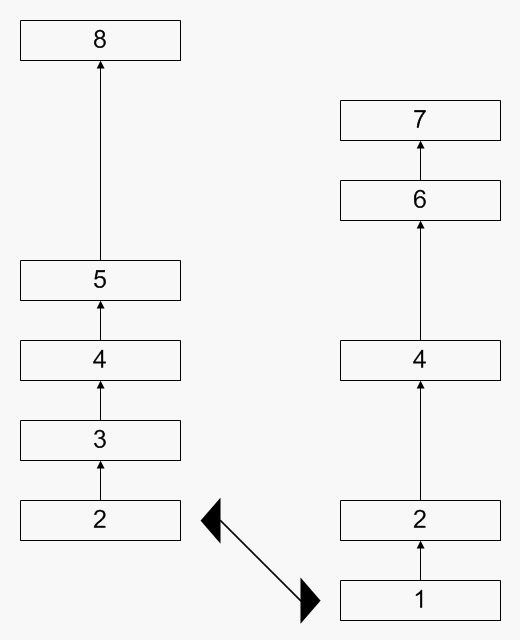So, we have the following pros:

• There is no need to hold either table in memory (unlike the hash joins)
• Performance can be comparable with hash joins if the records are pre-sorted (which is made possible by the underlying storage engine with an index)
• Supports the less than (`<`) and greater than (`>`) operators as well as the equality operator (`=`)

And the following cons:

• A quasilinear time complexity of O(Mlog(M) + Nlog(N)), which is better than the nested loop join (quadratic) but slower than the hash join (linear)
• If the records are not pre-sorted, this can be an expensive step
• A final sort might still be necessary (if the parent select orders the result set on different columns)
• The pointer code needs to handle duplicate values (see the animation above) which is complex and requires more code to implement than the nested loop algorithm or the hash join algorithm

## Making a Decision

We implemented a prototype for both algorithms and ran some microbenchmarking using the JMH tool.

Left Table Size Right Table Size Hash Join Sorted Merge (Presorted Inputs) Sorted Merge (Shuffled Inputs)
Small Large 0.354 ms/op 0.428 ms/op 3.318 ms/op
Large Small 1.056 ms/op 0.404 ms/op 3.315 ms/op

Here, ms/op is a measure of how many milliseconds per operation the query execution took.

From these results, we see that sort merge joins with shuffled input records perform the worst, by a considerable margin. This was expected.

Hash joins seem to perform slightly better than sort merge joins when the left table is smaller than the right table.

Based on these findings, and given that we wanted to optimize equi-joins, and so did not require support for less than (`<`) or greater than (`>`) join conditions, we decided to implement the hash-join algorithm.

## Preparation Work

CrateDB already implements push-down query optimization for joins. Essentially, this allows the CrateDB to filter, order, and limit records before the actual join operation, which eliminates unnecessary work during or after the join operation.

In particular, the `ORDER BY` operation can be pushed down to the left table (and removed from the parent select) if the `ORDER BY` columns refer to the left (outer) table of the join. This works because the order of the left table dictates the order of the emitted rows when performing a nested loop join.

For example, take this query:

``````  SELECT *
FROM t1
JOIN t2
ON t1.a = t2.b
ORDER BY t1.x
``````

This can be rewritten to push down the order by, like so:

``````SELECT *
FROM (
SELECT *
FROM t1
ORDER BY t1.x
) AS new_t1
JOIN t2
ON new_t1.a = t2.b
``````

Previously, this sort of query rewriting for the push-down optimizations took place during query analysis, before query planning.

This cannot work for hash joins, because the hash join algorithm does not preserve pushed-down order. Moreover, the decision to use a hash join algorithm doesn't even take place until query planning.

So we needed to change something here.

To prepare for the hash joins implementation, we had to move the push-down operation from the analyzer to the query planner.

After completing our changes, the query is planned as follows.

First, we create an unoptimized plan. This plan has a tree-like format.

Take this query:

``````  SELECT *
FROM t1
JOIN t2
ON t1.a = t2.b
ORDER BY t1.x
``````

The initial plan looks like this: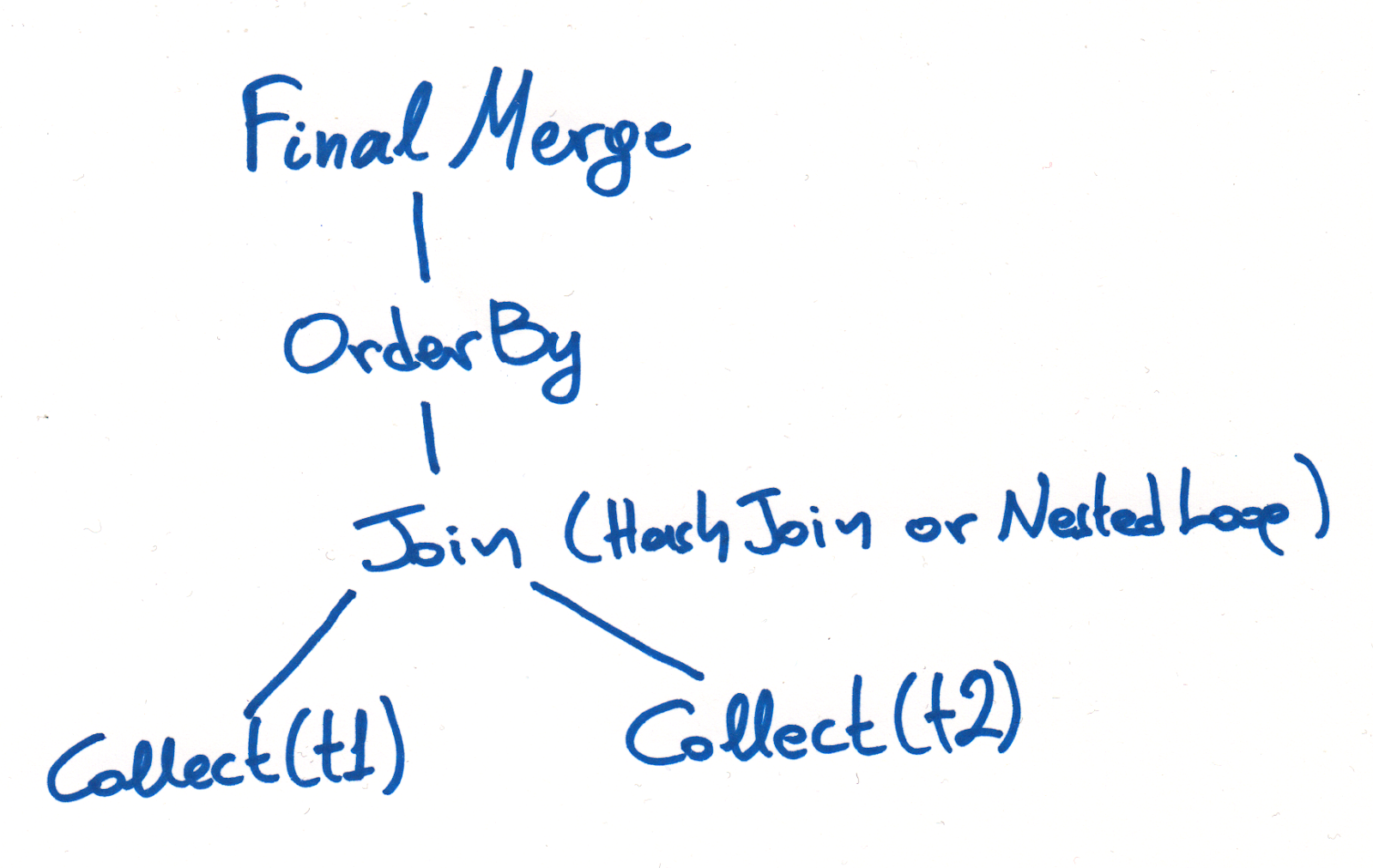Once we have this plan, we try to push down any operators that can be pushed down.

Pushing down operations allows CrateDB to perform operations earlier on in the query execution process, which is typically less expensive because there are fewer records to handle. If we push down an `ORDER BY`, for example, to the storage engine (Lucene), we can even take advantage of indexes to retrieve the rows pre-ordered.

In the case of our query above, the `Join` class object receives a method call with the `OrderBy` object as an argument. The specific `Join` subclass (either `HashJoin` or `NestedLoop`) can then decide for itself if it is possible to push down the `OrderBy` object.

If the `Join` is a `NestedLoop` subclass and the `OrderBy` object only contains symbols attached to the left (outer) table (`t1` in our case), then it can be pushed down and removed as a parent operator of the query.

When this happens, the `OrderBy` is pushed down to the collect phase (the point at which we fetch rows from the underlying storage engine) and an `OrderedCollect(t1)` operation is used instead of a `Collect(t1)` operation.

Also, because collection happens on multiple individual nodes across the cluster, a final `Merge` operation is added that ensures the intermediate result sets remain ordered when they are merged into a final result set.

The final plan looks like this: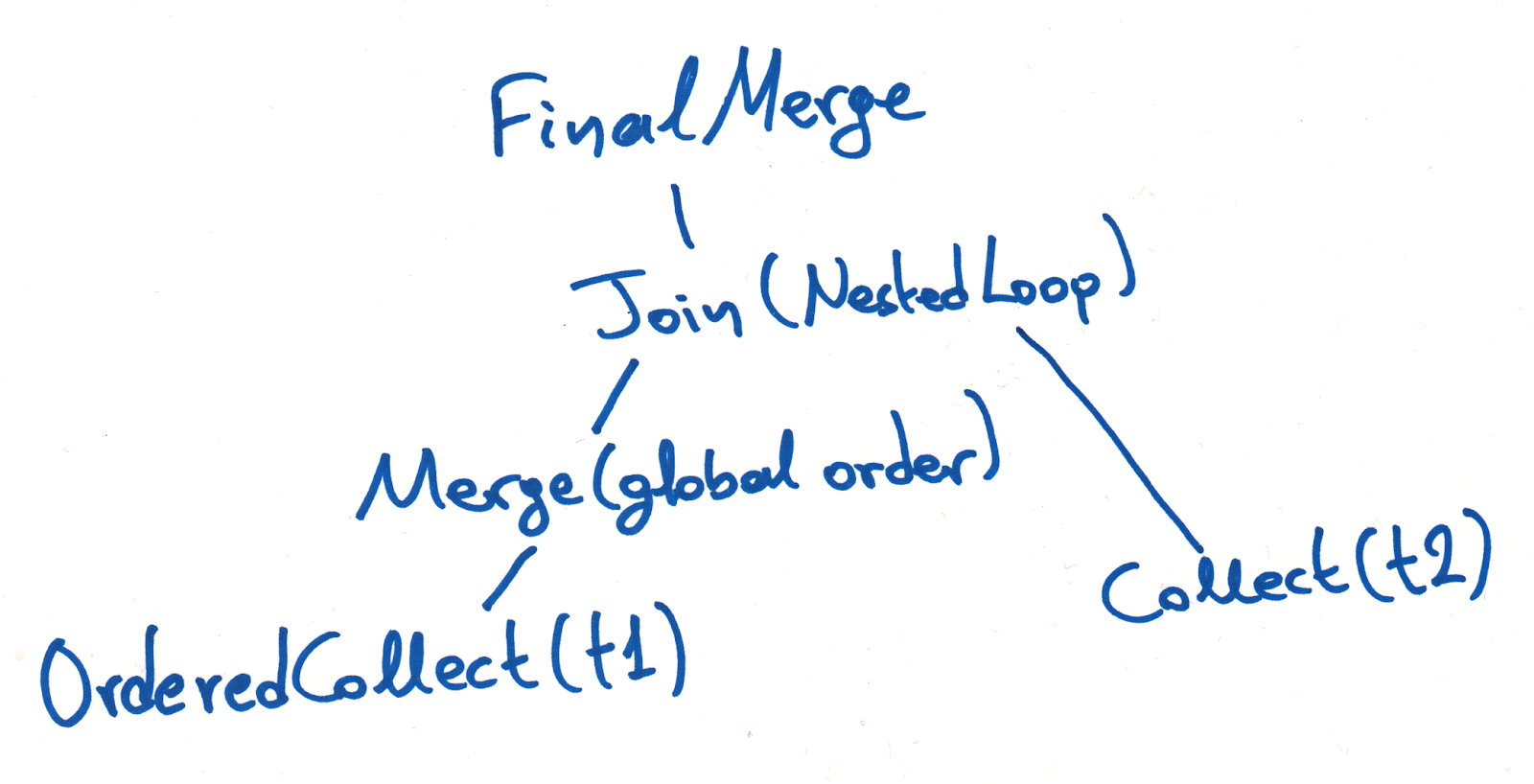## Implementation

For our initial implementation of the hash join algorithm, we decided to code for the simplest possible case. That is, when an equi-join is run on one node of the cluster, and one of the two tables can fit into memory.

We did go on to expand this functionality, but that will be the topic of two follow-up posts. For now, we are only going to consider the first iteration of this work.

### Detecting Hash Join Candidates

The first thing we needed to do was add a utility class that looks at the join condition of a join query and determines whether it is an equi-join, i.e., whether it uses a single equality operator (`=`) or multiple operators chained together with `AND` operators, and at least one of them is an equality.

This class must also ensure that the symbols on both sides of each equality operator refer only to one column. For example, `sqrt(t1.a + t2.b) = 10` means that the join is not an equi-join, because the left-hand side refers to two columns.

This class is, predictably enough, named `EquiJoinDetector`.

For example, these join conditions belong to equi-joins:

• `t1.a - 5 = t2.b + 10`
• `t1.a = t2.b AND sqrt(t1.x) = t2.y`
• `t1.a = t2.b AND t1.x > t2.y AND t1.i < t2.k`

And these do not:

• `log(t1.a + t2.b) = 2.5`
• `t1.a = t2.b OR t1.x = t2.y`
• `t1.a = sqrt(t2.b - t1.y)`

So if the join is an inner join and an equi-join condition is detected, the join pair can be executed with the hash join algorithm. This is because, as previously mentioned, we only implemented hash join for equi-joins in this batch of work.

### The Build Phase

The next step was to extract the columns that participate in the join condition so that we can determine where to apply the hash function.

To this end, we wrote a second utility class that processes the join condition and returns a HashMap. Each entry in the hash map uses a relation name (a table name or an alias) as a key and has a list of symbols of that relation participating in the join condition the corresponding value.

Here's a quick sketch of the build phase: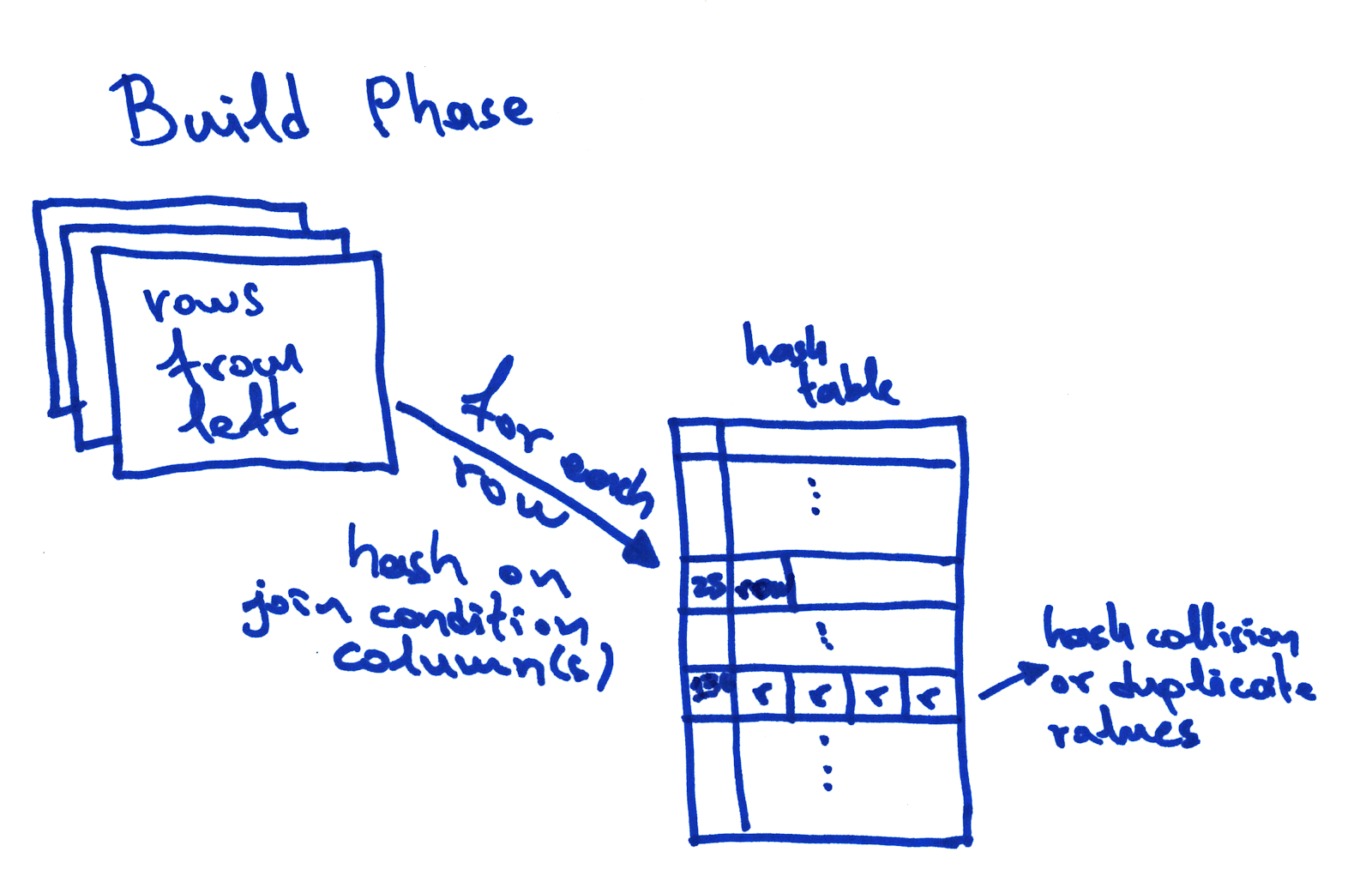### The Probe Phase

After implementing the build phase, it was time to implement the probe phase. The probe phase is the core of the hash join algorithm.

The `Join` operations are implemented with a set of classes that correspond to the different join types, for example, `CrossJoinNLBatchIterator`, `RightJoinNLBatchIterator`, and so on. (“NL” here stands for “nested loop.”) Each of them has two source iterators, the left and the right, which provide the rows of the left and right relation respectively.

For the new `HashInnerJoinBatchIterator` we had to introduce a new structure: a `HashMap` that holds the rows from the left relation along with the hash value calculated from the symbols of the relation that participate in the join condition.

After microbenchmarking two `HashInnerJoinBatchIterator` implementations we chose to go with `com.carrotsearch.hppc.IntObjectHashMap` instead of Java’s builtin `HashMap`, because it performs better.

However, we still have a problem.

It is possible that two or more rows produce an identical hash, which leads to a hash collision because hash maps only have one entry per hash. If we tried to write a second entry for the same hash value, we would overwrite the first entry.

The solution we adopted is known as a chained hash-table.

What this means is that each entry in the hash map is a list of rows, instead of a single row. This handles the case where multiple rows have the same hash. And when a hash only corresponds to a single row, the hash map has a list that only contains a single row.

### Tying It All Together

We now have all the necessary components to implement the algorithm.

Here's a high-level overview:

1. Build phase
1. Read all the rows from the left table one by one. For each row, calculate the hash and insert the row into the hash map.
2. Probe phase
1. Read all the rows from the right table one by one. For each row, calculate the hash and look it up in the hash map.
2. If no entry is found, then skip that right table row because it does not meet the join condition.
3. If an entry is found, then for each row of the left table in the list of the hash map entry, try to validate the join condition. If the join condition is true, emit the row. If not, skip that row and continue with the next row in the list.

Here's a quick sketch of the probe phase: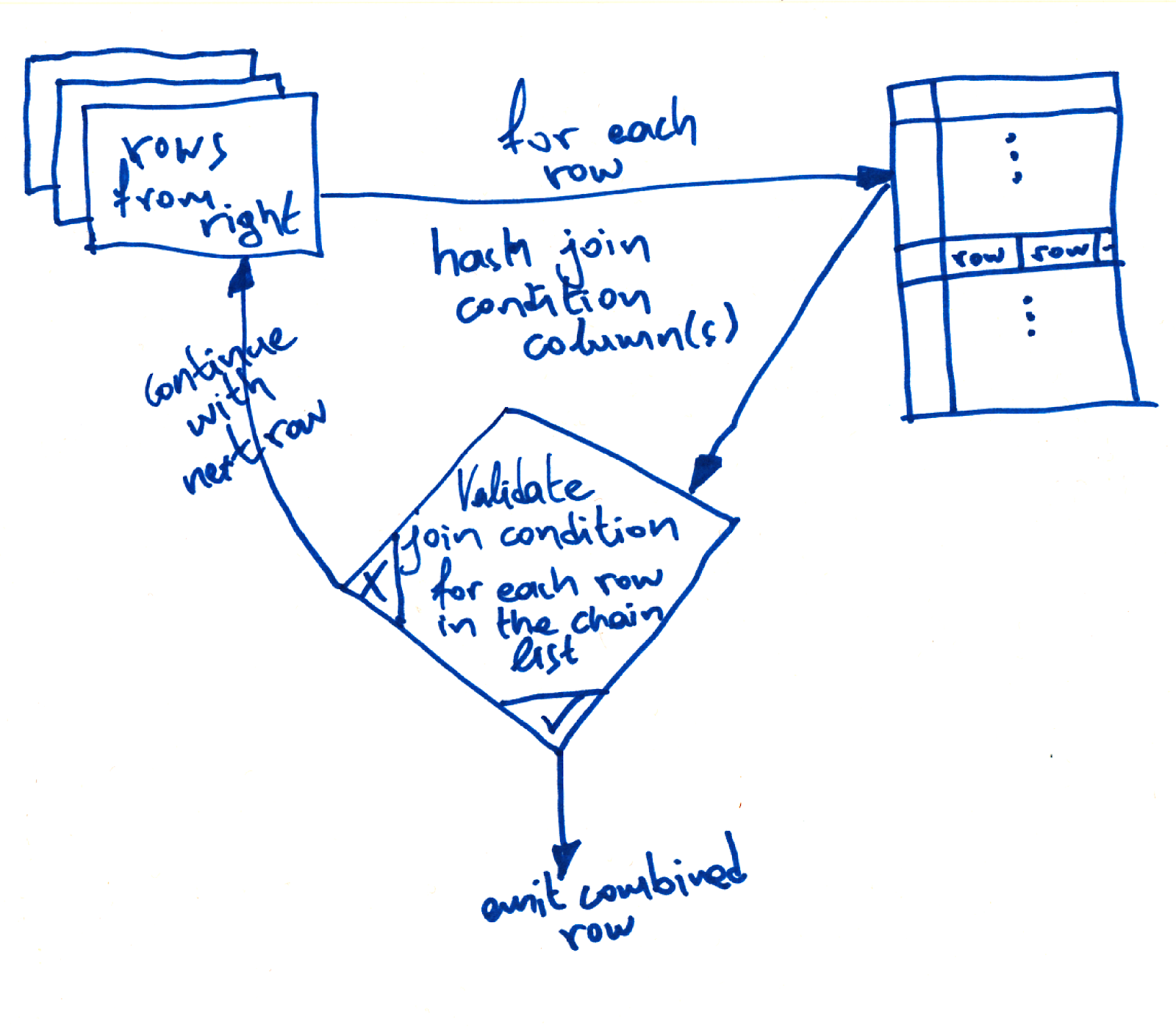The join condition validation is necessary because we're using a chained hash table. That means that even if we find an entry in the hash table, we cannot be sure which of the entry rows match the join condition until we check.

Something you might have noticed here is that to execute this algorithm we need to store all of the rows of the left (outer) table in a hash map. And HashMaps are held in memory. So as a result, we need to store the entire contents of the outer table in memory.

If the outer table is too large to fit in memory, this will result in an `OutOfMemory` exception, which will, in turn, kill the Java Virtual Machine (JVM), and ultimately, the running CrateDB node. Not a great outcome.

To prevent that we used the CircuitBreaker infrastructure.

What this means is that as we load the hash map up with rows, if we pass the configured memory limit, the circuit breaker is tripped, and as a result, the query is killed without bringing down the CrateDB node. A meaningful error message is also sent to the client.

## Results

We ran some full benchmarks on the completed feature.

These benchmarks confirm that the new hash join implementation has a significant impact on join performance when it is triggered.

For our benchmarks, we used a five node cluster, with 32 GB RAM (using a 16 GB Java heap) and 12 cores each.

Here's what we did:

• We created two tables, `t1` and `t2`, with five shards each
• We ran two join queries:
• One matching all rows, and
• One matching one-fifth of all rows
• We started with 10 thousand rows in each table and then increased that in increments up to one million rows
• We ran these query combinations with both the hash join algorithm and the nested loop algorithm
• Each query was executed multiple times and the average execution time was picked

Here's the query for matching all rows:

``````    SELECT count(*)
FROM t1
INNER JOIN t2
ON t1.id1 = t2.id1
``````

And here's the query for matching one fifth of all rows:

``````    SELECT count(*)
FROM t1
INNER JOIN t2
ON t1.id1 = t2.id1
AND t1.id2 = t2.id2
``````

These were the results we saw:

Query # Rows Nested Loop (execution time) Hash Join (execution time) Improvement
Match all 10,000 1.74110 ms 0.02187 ms 79x
Match one fifth 10,000 2.53793 ms 0.01922 ms 132x
Match all 20,000 6.35937 ms 0.02534 ms 250x
Match one fifth 20,000 9.73778 ms 0.02769 ms 351x
Match all 50,000 39.58197 ms 0.04643 ms 852x
Match one fifth 50,000 60.97238 ms 0.04494 ms 1,356x
Match all 100,000 158.63308 ms 0.07921 ms 2,002x
Match one fifth 100,000 242.13536 ms 0.07922 ms 3,056x
Match all 200,000 628.51570 ms 0.13737 ms 4,575x
Match one fifth 200,000 1025.84606 ms 0.13579 ms 7,554x
Match all 500,000 3986.60200 ms 0.35814 ms 11,131x
Match one fifth 500,000 N/A 0.35457 ms N/A
Match all 1,000,000 N/A 0.79487 ms N/A
Match one fifth 1,000,000 N/A 0.77291 ms N/A

Note: tests marked N/A ran for too long and were terminated.

What we see here:

• Nested loop query execution time increases quadratically.

That is, if you double the number of rows to return, the query execution time increases by approximately 4x.

• In stark contrast, the query time for hash joins increases linearly.

For example, in the table above, when the rows are doubled from 50,000 to 100,000, the query execution time only increases by around 1.7x.

We then repeated the experiment, using only queries that match all rows, but this time adding an `ORDER BY [...] LIMIT` to the query, like so:

``````
SELECT t1.id
FROM t1
INNEr JOIN t2
ON t1.id1 = t2.id1
ORDER BY t1.id1
LIMIT 100000
``````

This allows the nested loop algorithm to optimize the query by pushing the `ORDER BY` operation down to the storage level index.

These were the results:

# Rows Nested Loop
(execution time)
Hash Join
(execution time)
Improvement
10,000 1.77585 0.02675 66x
20,000 6.47108 0.04481 144x
50,000 40.16177 0.09729 412x
100,000 161.39823 0.18265 883x
200,000 635.32250 0.28421 2,235x
500,000 N/A 0.57170 N/A
1,000,000 N/A 1.12010 N/A

Note: tests marked N/A ran for too long and were terminated.

What this shows us is that the hash join algorithm still outperforms the nested loop algorithm, even when the nested loop algorithm can optimize with a push-down and the hash join algorithm cannot.

## Wrap Up

The work we did to implement hash joins as an alternative to our nested loop algorithm is an essential first step towards improved join performance.

Nested loop joins have a time complexity of O(M*N), and hash joins have a time complexity of O(M+N). This is a significant improvement, and the results of our benchmarks confirm this. We have seen performance improvements as substantial as 11,131x.

But wait, the headline on this blog post says we increased performance 23 thousand times. So what gives?

Well, we didn't stop here.

After completing this batch of work, we continued to work on join improvements. We implemented a block hash join algorithm, which addresses the memory limitations of the standard hash join algorithm. We then improved on this by distributing the block hash join execution across the entire cluster.

Tune in for parts two and three to learn how we effectively doubled the performance improvements shown in this post.